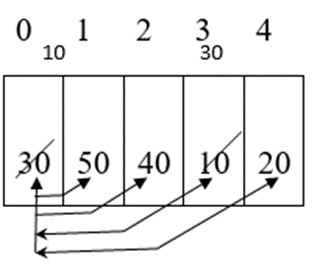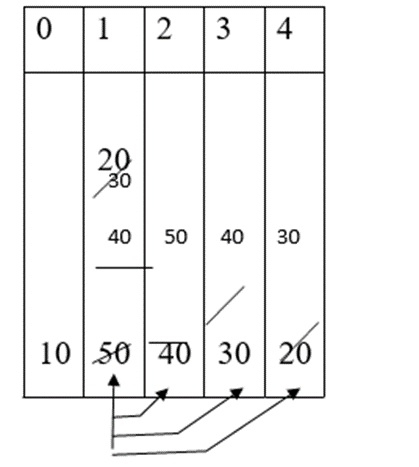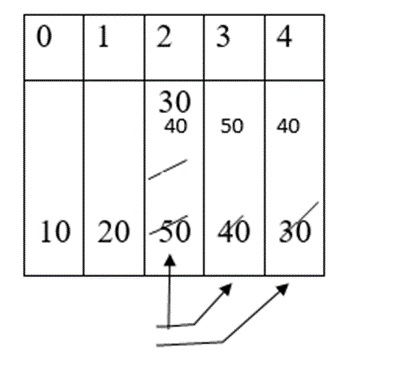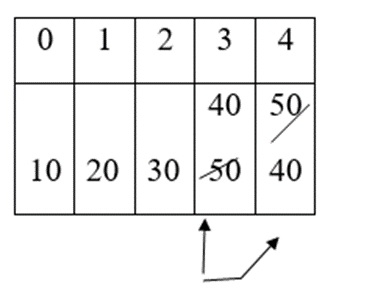# Explain the sorting techniques in C language

## Problem

What are the different sorting techniques in C Language? Explain any one sorting technique with an example.

## Solution

C language provides five sorting techniques, which are as follows −

• Bubble sort (or) Exchange Sort.
• Selection sort.
• Insertion sort (or) Linear sort.
• Quick sort (or) Partition exchange sort.
• Merge Sort (or) External sort.

## Bubble sort

It is the simplest sorting technique which is also called as an exchange sort.

### Procedure

• Compare the first element with the remaining elements in the list and exchange(swap) them if they are not in order.

• Repeat the same for other elements in the list until all the elements gets sorted.

30 50 40 10 20

Consider the elements given below −### First pass

Compare first element with the remaining elements.

a > a $\square$ $\square$30 >50 (F) $\square$ $\square$no exchange

a > a $\square$ $\square$ 30 >40 (F) $\square$ $\square$ no exchange

a > a $\square$ $\square$ 30 >10 (T) $\square$ $\square$ exchange

a > a $\square$ $\square$ 10>20 (F) $\square$ $\square$ no exchange

10 50 40 30 20

### Second Pass

Compare second element with the remaining elements.a > a $\square$ $\square$ 50 >40 (T) $\square$ $\square$ exchange

a > a $\square$ $\square$ 40 >30 (T) $\square$ $\square$ exchange

a > a $\square$ $\square$ 30 >20 (T) $\square$ $\square$ exchange

10 20 50 40 30

### Third Pass

Compare third element with the remaining elements.a > a $\square$ $\square$ 50 >40 (T) $\square$ $\square$ exchange

a > a $\square$ $\square$ 40 >30 (T) $\square$ $\square$ exchange

10 20 30 50 40

### Fourth Pass

Compare fourth element with the remaining elements.a > a $\square$ $\square$ 50 >40 (T) $\square$ $\square$ exchange

10 20 30 40 50

### Procedure

Refer the procedure for bubble sort as given below −

for (i=0; i<n-1; i++){
for (j=i+1; j<n; j++){
if (a[i] > a[j]){
t=a[i];
a[i] = a[j];
a[j] = t;
}
}
}

## Example

Following is the C program for bubble sorting technique −

Live Demo

#include<stdio.h>
int main(){
int a, i,j,n,t;
printf("enter the No: of elements in the list:");
scanf("%d", &n);
printf("enter the elements:");
for(i=0; i<n; i++){
scanf ("%d", &a[i]);
}
printf("Before bubble sorting the elements are:");
for(i=0; i<n; i++)
printf("%d \t", a[i]);
for (i=0; i<n-1; i++){
for (j=i+1; j<n; j++){
if (a[i] > a[j]){
t = a[i];
a[i] = a[j];
a[j] = t;
}
}
}
printf ("after bubble sorting the elements are:");
for (i=0; i<n; i++)
printf("%d\t", a[i]);
return 0;
}

## Output

When the above program is executed, it produces the following result −

enter the No: of elements in the list:
5
enter the elements:
12 11 45 26 67
Before bubble sorting the elements are:
12
11
45
26
67
after bubble sorting the elements are:
11 12 26 45 67